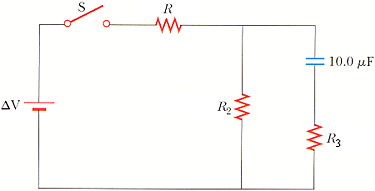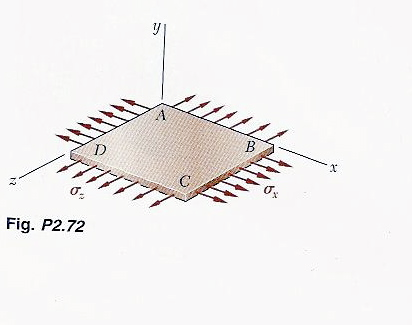Ask question

# A dead battery is charged by connecting it to the live battery ofanother car with jumper cables. Assume that V=9.5 V and R=0.90 Ohms. Determine the current in the starter. Your answer differs from the correct answerby orders of magnitude. A Determine the current in the dead battery. Your answer differs from the correct answerby orders of magnitude.# A dead battery is charged by connecting it to the live battery ofanother car with jumper cables. Assume that V=9.5 V and R=0.90 Ohms. Determine the current in the starter. Your answer differs from the correct answerby orders of magnitude. A Determine the current in the dead battery. Your answer differs from the correct answerby orders of magnitude.

Question
Otherasked 2021-04-02
A dead battery is charged by connecting it to the live battery ofanother car with jumper cables. Assume that V=9.5 V and R=0.90 Ohms.
Determine the current in the starter.
Your answer differs from the correct answerby orders of magnitude. A
Determine the current in the dead battery.
Your answer differs from the correct answerby orders of magnitude.

## Answers (1)2021-04-04
Assume current through starter be Is flowing downwards
Assume current through Dead battery be Id flowing downwards
So current through live battery is ($$\displaystyle{I}_{{s}}+{I}_{{d}}$$) flowing upwards
KVL for left loop -
$$\displaystyle+{9.5}{V}+{\left({0.9}\ {O}{h}{m}{s}\right)}{l}{d}-{\left({0.06}\ {O}{h}{m}{s}\right)}{l}{s}={0}$$
KVL for the big loop
$$\displaystyle+{12}{V}-{\left({0.01}\ {O}{h}{m}{s}\right)}{\left({l}{s}+{l}{d}\right)}-{\left({0.06}\ {O}{h}{m}{s}\right)}{l}{s}={0}$$
Solving these teo equation, we get
$$\displaystyle{l}_{{s}}={0.865}\ {A}$$
$$\displaystyle{l}_{{d}}={171.305}\ {A}$$

### Relevant Questionsasked 2021-05-09
The dominant form of drag experienced by vehicles (bikes, cars,planes, etc.) at operating speeds is called form drag. Itincreases quadratically with velocity (essentially because theamount of air you run into increase with v and so does the amount of force you must exert on each small volume of air). Thus
$$\displaystyle{F}_{{{d}{r}{u}{g}}}={C}_{{d}}{A}{v}^{{2}}$$
where A is the cross-sectional area of the vehicle and $$\displaystyle{C}_{{d}}$$ is called the coefficient of drag.
Part A:
Consider a vehicle moving with constant velocity $$\displaystyle\vec{{{v}}}$$. Find the power dissipated by form drag.
Express your answer in terms of $$\displaystyle{C}_{{d}},{A},$$ and speed v.
Part B:
A certain car has an engine that provides a maximum power $$\displaystyle{P}_{{0}}$$. Suppose that the maximum speed of thee car, $$\displaystyle{v}_{{0}}$$, is limited by a drag force proportional to the square of the speed (as in the previous part). The car engine is now modified, so that the new power $$\displaystyle{P}_{{1}}$$ is 10 percent greater than the original power ($$\displaystyle{P}_{{1}}={110}\%{P}_{{0}}$$).
Assume the following:
The top speed is limited by air drag.
The magnitude of the force of air drag at these speeds is proportional to the square of the speed.
By what percentage, $$\displaystyle{\frac{{{v}_{{1}}-{v}_{{0}}}}{{{v}_{{0}}}}}$$, is the top speed of the car increased?
Express the percent increase in top speed numerically to two significant figures.asked 2021-03-21
In the figure below, suppose that the switch has been closed for a length of time sufficiently long enough for the capacitor to become fully charged. (R = 13.0 k, R2 = 20.0 k, R3 = 5.00 k, ΔV = 9.30 V)(a) Find the steady-state current in each resistor.
(b) Find the charge on the capacitor.asked 2021-05-20
Assume that a ball of charged particles has a uniformly distributednegative charge density except for a narrow radial tunnel throughits center, from the surface on one side to the surface on the opposite side. Also assume that we can position a proton any where along the tunnel or outside the ball. Let $$\displaystyle{F}_{{R}}$$ be the magnitude of the electrostatic force on the proton when it islocated at the ball's surface, at radius R. As a multiple ofR, how far from the surface is there a point where the forcemagnitude is 0.44FR if we move the proton(a) away from the ball and (b) into the tunnel?asked 2021-04-13
As depicted in the applet, Albertine finds herself in a very odd contraption. She sits in a reclining chair, in front of a large, compressed spring. The spring is compressed 5.00 m from its equilibrium position, and a glass sits 19.8m from her outstretched foot.
a)Assuming that Albertine's mass is 60.0kg , what is $$\displaystyle\mu_{{k}}$$, the coefficient of kinetic friction between the chair and the waxed floor? Use $$\displaystyle{g}={9.80}\frac{{m}}{{s}^{{2}}}$$ for the magnitude of the acceleration due to gravity. Assume that the value of k found in Part A has three significant figures. Note that if you did not assume that k has three significant figures, it would be impossible to get three significant figures for $$\displaystyle\mu_{{k}}$$, since the length scale along the bottom of the applet does not allow you to measure distances to that accuracy with different values of k.asked 2021-02-14
The plane of a rectangular coil, 5.0-cm by 8.0-cm, is perpendicular to the direction of a magnetic field "B". If the coil has 75 turns and a total resistance of 8.0 Ohms, at what rate must the magnitude of "B" change to induce a current of 0.10 amps in the windings of the coil?asked 2021-05-05
A person accidentally leaves a car with the lights on. If eachof the two headlights uses 43 W andeach of the two taillights 7.0 W,for a total of 1.0E2 W, how longwill a fresh 12-V battery last if it is rated at 8.0E1 A·h?
Assume the full 12 V appears across each bulb.asked 2021-04-25
The unstable nucleus uranium-236 can be regarded as auniformly charged sphere of charge Q=+92e and radius $$\displaystyle{R}={7.4}\times{10}^{{-{15}}}$$ m. In nuclear fission, this can divide into twosmaller nuclei, each of 1/2 the charge and 1/2 the voume of theoriginal uranium-236 nucleus. This is one of the reactionsthat occurred n the nuclear weapon that exploded over Hiroshima, Japan in August 1945.
A. Find the radii of the two "daughter" nuclei of charge+46e.
B. In a simple model for the fission process, immediatelyafter the uranium-236 nucleus has undergone fission the "daughter"nuclei are at rest and just touching. Calculate the kineticenergy that each of the "daughter" nuclei will have when they arevery far apart.
C. In this model the sum of the kinetic energies of the two"daughter" nuclei is the energy released by the fission of oneuranium-236 nucleus. Calculate the energy released by thefission of 10.0 kg of uranium-236. The atomic mass ofuranium-236 is 236 u, where 1 u = 1 atomic mass unit $$\displaystyle={1.66}\times{10}^{{-{27}}}$$ kg. Express your answer both in joules and in kilotonsof TNT (1 kiloton of TNT releases 4.18 x 10^12 J when itexplodes).asked 2021-03-28The homogeneous plate ABCD is subjected to a biaxial loading asshown. It is known that $$\displaystyle\sigma_{{z}}=\sigma_{{o}}$$ and that the change in length of the plate in the x direction must be zero, that is $$\displaystyle\epsilon_{{x}}={0}$$. Denoting E the modulus of elasticity and by v Poisson's ratio, determine (a) the required magnitude of $$\displaystyle\sigma_{{x}}$$, and (b) the ratio $$\displaystyle{\frac{{\sigma_{{o}}}}{{\epsilon_{{z}}}}}$$asked 2021-04-15
A car initially traveling eastward turns north by traveling in a circular path at uniform speed as in the figure below. The length of the arc ABC is 235 m, and the car completes the turn in 33.0 s. (Enter only the answers in the input boxes separately given.)
(a) What is the acceleration when the car is at B located at an angle of 35.0°? Express your answer in terms of the unit vectors $$\displaystyle\hat{{{i}}}$$ and $$\displaystyle\hat{{{j}}}$$.
1. (Enter in box 1) $$\displaystyle\frac{{m}}{{s}^{{2}}}\hat{{{i}}}+{\left({E}{n}{t}{e}{r}\in{b}\otimes{2}\right)}{P}{S}{K}\frac{{m}}{{s}^{{2}}}\hat{{{j}}}$$
(b) Determine the car's average speed.
3. ( Enter in box 3) m/s
(c) Determine its average acceleration during the 33.0-s interval.
4. ( Enter in box 4) $$\displaystyle\frac{{m}}{{s}^{{2}}}\hat{{{i}}}+$$
5. ( Enter in box 5) $$\displaystyle\frac{{m}}{{s}^{{2}}}\hat{{{j}}}$$asked 2021-04-21
Car 1 has a mass of m1 = 65 ❝ 103 kg and moves at a velocity of v01 = +0.81 m/s. Car 2, with a mass of m2 = 92 ❝ 103 kg and a velocity of v02 = +1.2 m/s, overtakes car 1 and couples to it. Neglect the effects of friction in your answer.
(a) Determine the velocity of their center of mass before the collision m/s
(b) Determine the velocity of their center of mass after the collision m/s
(c) Should your answer in part (b) be less than, greater than, or equal to the common velocity vf of the two coupled cars after the collision? less than greater than equal to
...